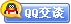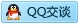##用户名 Email 自动登录 找回密码 密码 会员注册
 VIP会员，3年作业免费下 ！ 奥鹏作业，奥鹏毕业论文检测 新手作业下载教程，充值问题 没有找到答案，请在此处留言！ 2019年12月最新全国统考资料 投诉建议，加盟合作！奥鹏课程积分软件(ver:3.1)

# 东大19秋学期《电路原理》在线平时作业3（参考）发表于 2019-9-7 03:01:47 | 显示全部楼层 |阅读模式【奥鹏】[东北大学]19秋学期《电路原理》在线平时作业3 试卷总分:100    得分:100 第1题,图中，L=20mH, C=50pF, R=1kW, ù=10D00 V。电路的谐振频率w0为(       )弧度/秒 。A 106   其它      B 159155       C A、A B、B C、C D、D 正确答案: 第2题,图中t30时iL(t) 为(      )。A iL(t)=3e-4t     B iL(t)=3-3e-4t    C 其它 A、A B、B C、C D、D 正确答案: 第3题,图中，L=10mH，C=10mF，ù=1D00 V。电路的电容电流有效值IC为(      )安。A 105   其它      B 0       C A、A B、B C、C D、D 正确答案: 第4题,8-1 图中所示电路L=0.2H,R=2Ω,求冲击响应i为（      ） 。 A 5e-t1(t)A               B 0                  C其它 A、A B、B C、C D、D 正确答案: 第5题,图中所示电路C=0.1F,R=10Ω,求冲击响应i为（      ） 。 A 0.1d(t)-0.1e-t1(t)A        B -0.1e-t1(t)A                C其它 A、A B、B C、C D、D 正确答案: 第6题,R=15Ω, L=0.3mH, C=0.2mF,U=5V,f=3*104Hz .电压UL=8.42V  。（    ） A、错误 B、正确 正确答案: 第7题,三角形联接的三相电路可以是三线制也可以是四线制。(         ) A、错误 B、正确 正确答案: 第8题,图中 R1=6Ω, R2=4 Ω, R3=3 Ω, US1=12V, US2=24V。则US2输出的电功率为240W。(       ) A、错误 B、正确 正确答案: 第9题,图中电流I1=6A。(      ) A、错误 B、正确 正确答案: 第10题,电路中的开关断开已经很久，t=0时闭合开关，电压u1(0)=1V 。(      ) A、错误 B、正确 正确答案: 第11题,已知 R=1Ω, C=1F, a=2。 e(t)=d(t)V时的uC(t)=e-t 1(t)V（      ） 。 A、错误 B、正确 正确答案: 第12题,图中 R1=6Ω, R2=4 Ω, R3=3 Ω, US1=12V, US2=24V。则电流I1 =2A.(      ) A、错误 B、正确 正确答案: 第13题,图中 R1=6Ω, R2=4 Ω, R3=3 Ω, US1=12V, US2=24V。则电阻R1消耗的电功率为24W。(       ) A、错误 B、正确 正确答案: 第14题,图中 US=10V, R=10Ω, IS=1A。则电流=-1A 。(       ) A、错误 B、正确 正确答案: 第15题,图中复阻抗Z=R+jwL-j[1/(wC)]。(    ) A、错误 B、正确 正确答案: 第16题,图示电路，t=0 时将开关K闭合，t0时电路已达稳态，电压uc(0)=10V 。(      ) A、错误 B、正确 正确答案: 第17题,F(S)=(S+8)/[S(S+2)2]的原函数f(t)=[2-(2+3t)e-2t]1(t)（      ）。 A、错误 B、正确 正确答案: 第18题,虚数单位j在相量中表示+90度，一个相量乘上j表示这个相量被加上90度。(       ) A、错误 B、正确 正确答案: 第19题,换路定律是指换路瞬间所有元件的电压与电流不跳变，即u(0+)=u(0-)和i(0+)=i(0-)。(      ) A、错误 B、正确 正确答案: 第20题,R=15Ω, L=0.3mH, C=0.2mF,U=5V,f=3*104Hz .电压UC=3.95V  。（    ） A、错误 B、正确 正确答案:

### 本帖子中包含更多资源

x
 您需要登录后才可以回帖 登录 | 会员注册 本版积分规则 回帖并转播 回帖后跳转到最后一页客服一客服二客服三客服四微信客服扫一扫